4 Ленц ережесі. Өздік индукция. Индуктивтілік. Қосымша 3
Оценка 4.7

# 4 Ленц ережесі. Өздік индукция. Индуктивтілік. Қосымша 3

Оценка 4.7
doc
07.05.2020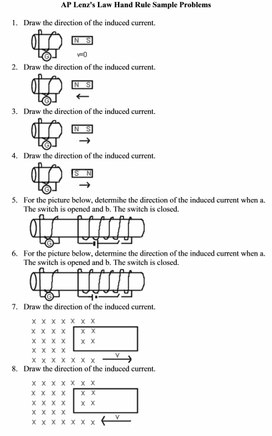4 Ленц ережесі. Өздік индукция. Индуктивтілік. Қосымша 3.doc

AP Lenz's Law Hand Rule Sample Problems

1. Draw the direction of the induced current.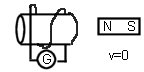2. Draw the direction of the induced current.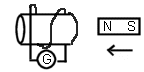3. Draw the direction of the induced current.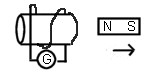4. Draw the direction of the induced current.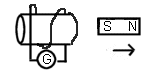5. For the picture below, determihe the direction of the induced current when a. The switch is opened and b. The switch is closed.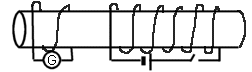6. For the picture below, determine the direction of the induced current when a. The switch is opened and b. The switch is closed.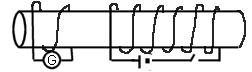7. Draw the direction of the induced current.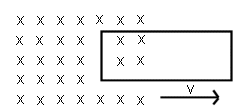8. Draw the direction of the induced current.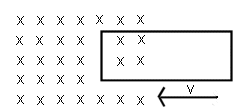9. For the picture below, what is the direction of the current through R caused by current i in the wire below the loop?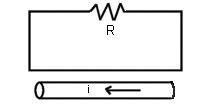10. What is the direction of the current in the loop pictured below?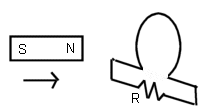11. The conducting bars are moved to the right, from position A to B, in the picture below. The uniform magnetic field is directed outward. What is the direction of the induced current in the loop?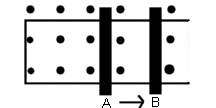12. The conducting bars are moved to the right, from position A to B, in the picture below. The uniform magnetic field is directed inward. What is the direction of the induced current in the loop?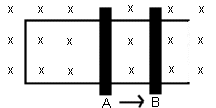AP Electromagnetic Induction Sample Problems

http://www.pschweigerphysics.com/APinducprob.html

1. Consider the arrangement shown below. Assume that R=6 W and l = 1.2 m. There is a uniform 2.5 T magnetic field directed into the page. A 0.5 A current is produced in the resistor. What voltage is induced in the bar? At what speed should the bar be moved to produce a current of 0.5 A in the resistor? At what rate is work done to keep the bar moving at this speed? Ans: 3 V; 1.0 m/s; 1.5 W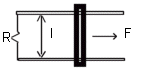2. Two long straight wires are separated by 0.120 m. The wires carry currents of 8 A in opposite directions; the current in the wire on the left is down and that in the wire on the right is up. Find the magnitude and direction of the net magnetic fields at points A, B, and C as shown. Ans: 4.3 x 10-5 T, out, at A; 5.3 x 10-5 T, in, at B; 6 x 10-5 T, in, at C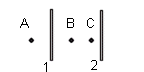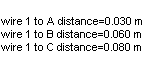1. A square, single-turned coil 0.20 m on a side is placed with its plane perpendicular to a constant magnetic field. An emf of 18 mV is induced when he area of the coil decreases at a rate of 0.10 m2/s. What is the magnitude of the magnetic field? Ans: 0.18 T
2. The sliding bar in the figure below has a length of 0.50 m and moves at 2 m/s in a magnetic field of 0.25 T. Find the induced voltage in the moving rod. If the resistance in the circuit is 0.50 W, find the current in the circuit. Find the amount of energy dissipated by the resistor in one second. The source of energy that is dissipated in the resistor is some external agent that keeps the bar moving at a constant speed of 2 m/s by exerting an applied force F. Find the value of F. Ans: 0.25 V; 0.50 A; 0.13 J; 0.063 N3. A 130 turn coil with a diameter of 2.1 cm is placed in a 0.0415 T magnetic field. What is the magnetic flux through the coil? What is the value of the induced emf if the magnetic field is reduced to zero in 50 msec? IF the coil has a resistance of 4 W, what current flows through the coil as the magnetic field is reduced? Ans: 1.44 x 10-5 Wb; 0.0375 V; 0.0093 A

Скачать файл